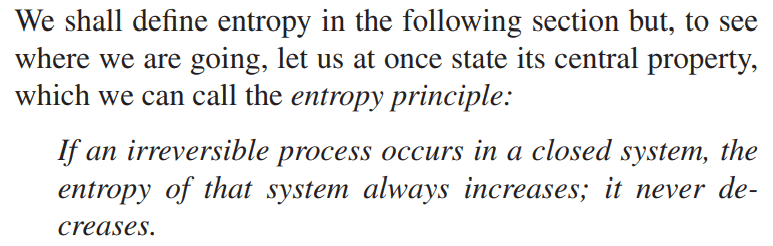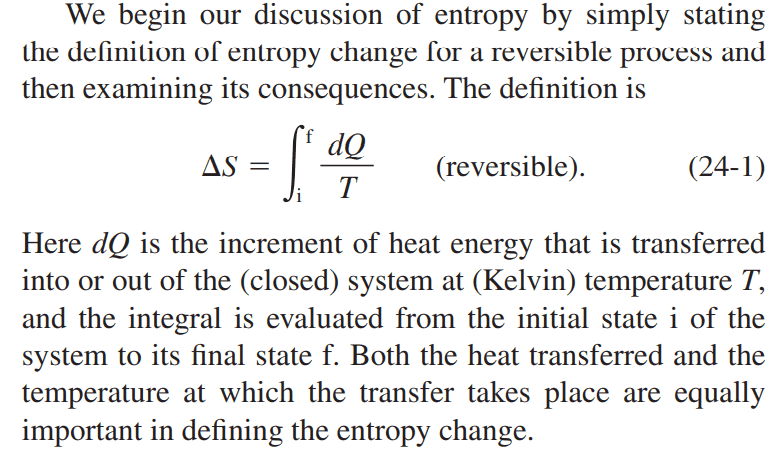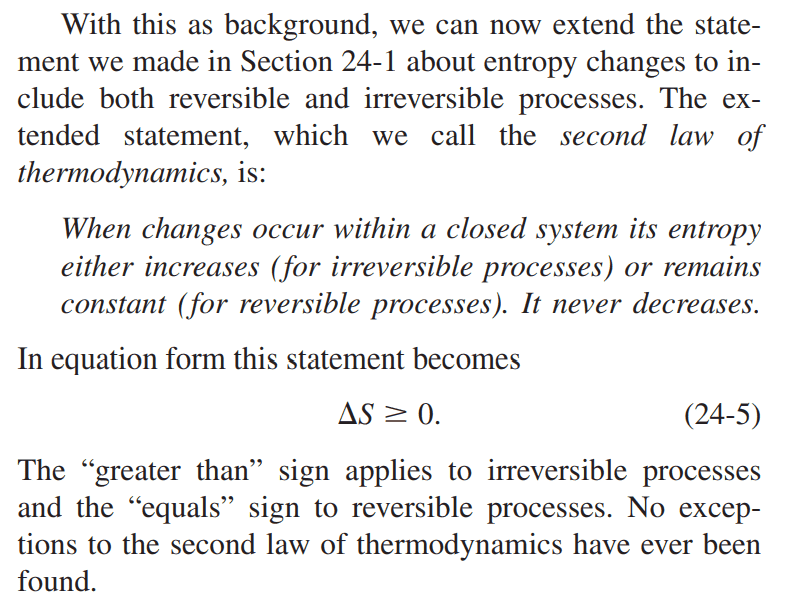• Afo
The answer to the OP is quite boring; reversible processes are defined as those for which the entropy of the system remains constant.This is not true; ##\delta Q## is not necessarily zero in a reversible process. (For example, consider isothermal expansion.)f

#### Afo

Homework Statement:: Why is the entropy of a closed system constant in a reversible process, and not related by ##\Delta S = \int_{i}^{f}\frac{dQ}{T}## (See below for the question in more details)
Relevant Equations:: ##\Delta S = \int_{i}^{f}\frac{dQ}{T}##

I am reading chapter 24 of Physics 1 by Halliday, Resnick, and Krane about the second law of thermodynamics and entropy.

Here's what I know:
1. A closed system is where there is no mass transfer between the environment, but there can be energy transfer as heat.
2. An isolated system is where there is no energy and mass transfer.
3. The entropy of a closed system increases in an irreversible process.4. The change of entropy, ##\Delta S## for a reversible process in a closed system is defined as ##\Delta S = \int_{i}^{f}\frac{dQ}{T}##The Question:

But then, the second law of thermodynamics states that the entropy remains constant for reversible processes. It's a contradiction since previously, the change in entropy was defined in the integral stated above.I think for reversible processes the total variation of entropy is equal to zero because dQ = 0. I think it's like pumping your bike in two different ways :
1) You do it very fast, entropy is generated and heat is also generated, and you can feel it by touching your bike pump. This process is irreversible.
2) If you decide to pump your bike slowly, "no" heat will be generated, and the variation of entropy will be equal to zero. This is a "reversible" process. (real reversible processes aren't real).

The integral there is just to show that entropy only depends on the final and initial state of your system, and since they're "identical", the variation of entropy in reversible processes will be equal to 0.

But I'm not too sure though, I'm only a student, someone please fact check.

I think for reversible processes the total variation of entropy is equal to zero because dQ = 0. I think it's like pumping your bike in two different ways :
This is not true; ##\delta Q## is not necessarily zero in a reversible process. (For example, consider isothermal expansion.)

The answer to the OP is quite boring; reversible processes are defined as those for which the entropy of the system remains constant.

••vanhees71 and duchuy
This is not true; ##\delta Q## is not necessarily zero in a reversible process. (For example, consider isothermal expansion.)

The answer to the OP is quite boring; reversible processes are defined as those for which the entropy of the system remains constant.
But doesn't isothermal mean constant temperature? So at constant T, if the variation of entropy is 0, dQ must also be equal to zero no? :(

This is not true; ##\delta Q## is not necessarily zero in a reversible process. (For example, consider isothermal expansion.)

The answer to the OP is quite boring; reversible processes are defined as those for which the entropy of the system remains constant.
Yes, but that is not exactly consistent with "the definition of entropy change for a reversible process" is the integral in 24-1. Should it say that is the definition of the entropy change for any process, then define a reversible process as one in which the change is zero?

•Afo
Correct me If I am wrong. I think the book meant to say that the entropy change of a closed system is ##\Delta S = \int_{i}^{f} \frac{dQ}{T}## while the second law of thermodynamics states that the entropy change of an isolated system is ##0## for reversible processes. While for irreversible processes in an isolated system, ##\Delta S >0##.

Yes, but that is not exactly consistent with "the definition of entropy change for a reversible process" is the integral in 24-1. Should it say that is the definition of the entropy change for any process, then define a reversible process as one in which the change is zero?
I think this is consistent after reading other books

If Haliday, Resnick, and Krane said all those things, then they are incorrect. This all has to do with the definition of a closed system and the definition of an isolated system. I think they meant to say that a closed system is one which cannot exchange mass with its surroundings but can exchange both heat and work, and that an isolated system is one that can exchange neither mass, heat, nor work with its surroundings. So for an isolated system, $$\Delta S\geq 0$$and for a closed system $$\Delta S\geq\int_{init}^{fin}{\frac{dQ}{T}}$$In both cases, the equal sign applies to a reversible path between the initial and final states of the system and the > sign applies to an irreversible path.

•BvU, Lord Jestocost, vanhees71 and 1 other person
Yes, but that is not exactly consistent with "the definition of entropy change for a reversible process" is the integral in 24-1. Should it say that is the definition of the entropy change for any process, then define a reversible process as one in which the change is zero?

In a reversible change, the entropy of an isolated system is constant. The entropies of its interacting sub-systems are not necessarily constant.

Usually, we will consider a system to be interacting with its surroundings (exchanging heat, work, etc.). Their union (the universe) may be considered an isolated system. The increment of the entropy of the system is related to the heat supplied to the system by the general inequality \begin{align*}
\delta S_{\mathrm{sys}} \geq \dfrac{\delta Q}{T_{\mathrm{surr}}}
\end{align*}due to Clausius. Equality holds for a reversible process. It should be emphasised that, for the surroundings, one may write\begin{align*}
\delta S_{\mathrm{surr}} = \dfrac{-\delta Q}{T_{\mathrm{surr}}}
\end{align*}The entropy change of the universe for this process is\begin{align*}
\delta S \equiv \delta S_{\mathrm{sys}} + \delta S_{\mathrm{surr}} \geq 0
\end{align*}where, again, equality holds only if the process is reversible.

But doesn't isothermal mean constant temperature? So at constant T, if the variation of entropy is 0, dQ must also be equal to zero no? :(

This is not the correct conclusion for the gas. If one allows the gas to expand slowly and isothermally, then the internal energy is constant and the first law implies\begin{align*}
dU = dQ - pdV = 0 \implies dQ = pdV
\end{align*}Assuming the ideal gas law, then ##p = nRT/V## and the heat absorbed by the gas must be \begin{align*}
Q = \int dQ = nRT \int \dfrac{dV}{V} = nRT \log{ \left( \dfrac{V_2}{V_1} \right)}
\end{align*}Meanwhile the entropy change of the gas is ##\Delta S_{\mathrm{sys}} = Q/T = nR \log{ \left( \dfrac{V_2}{V_1} \right)}##. This is offset by a decrease ##\Delta S_{\mathrm{surr}} = -\Delta S_{\mathrm{sys}}## in the entropy of the surroundings, so that the entropy of the universe remains constant.

•Afo and vanhees71
Thank you [B]ergospherical[/B] and [B]ergospherical[/B]. I have cleared all of my doubts. I believe that the sentences (in the book) are badly worded, and not because of the authors' knowledge. After reinterpreting it, I found out what they are trying to say.